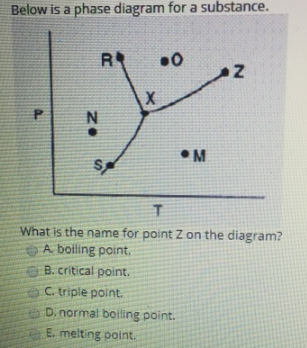# Problem: Below is a phase diagram for a substance. What is the name for point Z on the diagram? (A) boiling point. (B) critical point. (C) triple point. (D) normal boiling point. (E) melting point.

###### FREE Expert Solution

Point Z is considered the critical (supercritical) point which is the condition where its hard to distinguish if###### Problem Details

Below is a phase diagram for a substance.

What is the name for point Z on the diagram?

(A) boiling point.

(B) critical point.

(C) triple point.

(D) normal boiling point.

(E) melting point.